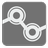Expected Dice
Simple+
English

For this task, you'll be playing some classic tabletop games. Or rather, you're going to be, but first you'd like to know more about the probabilities involved in dice rolling.

Many board games fall under the category of "roll and move" games. In these games, you roll some dice and move that many spaces forward. You might then move forward or backwards a number of spaces depending on which space you land on.

Given a description of the board and the dice used, you want to work out what the expected number of moves would be to reach a target square. For this task, all the boards under consideration are circular and when moving off one end you simply continue from the other. A board is represented as a list of integers. Each cell number means how many cells to move (positive for forward, negative for backwards) after landing on that cell. You always start from the first cell (index 0) and the starting and target squares will not require any further movement after landing on them.

For example: If we have one 4-sided die, the simple board [0,0,0,0] and any target square, then you have a 1/4 chance of reaching the target on every roll, so on average it will take 4 rolls. The result should be given with one digit precision as ±0.1.

Input: A number of dice to roll as an integer, the number of sides on each die as an integer, a target space as an integer and a board as a list of integers.

Output: The expected number of moves needed to reach the target space starting from space 0, correct to 1 digit after the decimal place. If it takes 33 1/3 moves, you should return 33.3. All test cases have a finite expected number of moves.

Example:

```expected(1, 4, 3, [0, 0, 0, 0]) == 4.0   # On these first three, you have a 1/4 chance of reaching the target
expected(1, 4, 1, [0, 0, 0, 0]) == 4.0   # on every roll, so on average it will take 4 rolls.
expected(1, 4, 3, [0, -1, -2, 0]) == 4.0
expected(1, 4, 3, [0, 2, 1, 0]) == 1.3   # You have a 3/4 chance of reaching the exit and 1/4 chance of ending up where you started
expected(1, 6, 1,  * 10) == 8.6
expected(2, 6, 1,  * 10) == 10.2
```

How it is used: Some of the methods for solving this task can be used to tackle a wide variety of other problems.

Preconditions:
1 ≤ dice_number ≤ 3
2 ≤ sides ≤ 10
0 < target < len(board)
4 ≤ len(board) ≤ 30
board == 0
board[target] == 0
all(board[(i + board[i]) % len(board)] == 0 for i in range(len(board)))